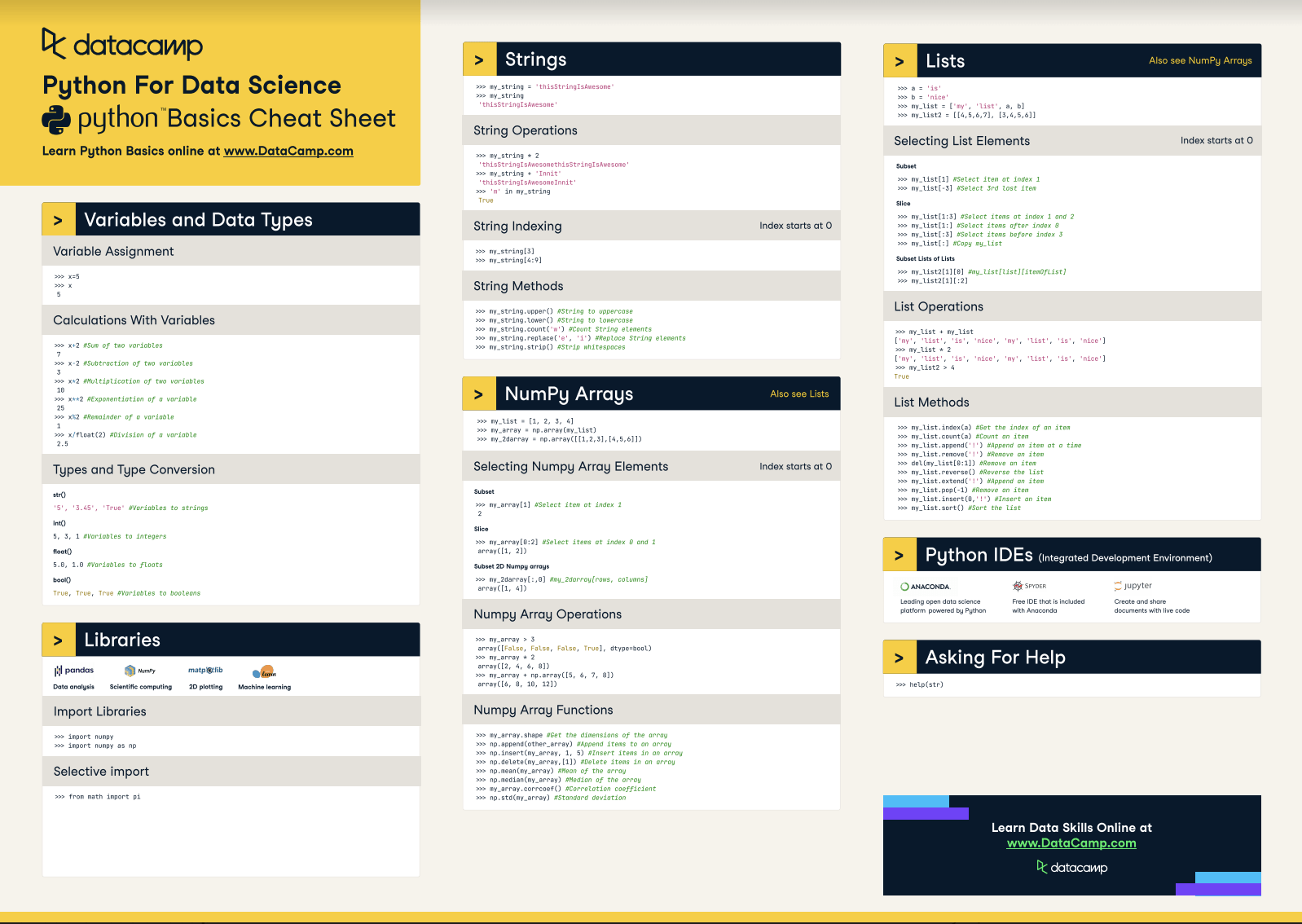# Python for Data Science - A Cheat Sheet for Beginners

This handy one-page reference presents the Python basics that you need to do data science

54% of the respondents from the O'Reilly Data Science Salary Survey indicated that they used Python as a data science tool. Nobody can deny that Python has been on the rise in the data science industry and it certainly seems that it's here to stay.

This rise in popularity in the industry, the long gone infancy of Python packages for data analysis, the low and gradual learning curve and the fact that it is a fully fledged programming language are only a couple of reasons that make Python an exceptional tool for data science.

Although Python is a very readable language, you might still be able to use some help.

That's enough reason for DataCamp to make a Python cheat sheet for data science, especially for beginners. It can serve as a quick reference for those of you who are just beginning their data science journey or it can serve as a guide to make it easier to learn about and use Python.

This cheat sheet is free additional material that complements DataCamp's Intro to Python for Data Science course, where you learn by doing.(Above is the printable version of this cheat sheet)

This Python cheat sheet will guide you through variables and data types, Strings, Lists, to eventually land at the fundamental package for scientific computing with Python, Numpy.

## Libraries

### Import libraries

 `import numpy` `import numpy as np`

### Selective import

 `from math import pi`

 `>>> help(str)`

## Variables and Data Types

### Variable Assignment

 `>>> x=5` `>>> x` `5`

### Calculations With Variables

 Sum of two variables `>>> x+2` `7` Subtraction of two variables `>>> x-2` `3` Multiplication of two variables `>>> x*2 ` `10` Exponentiation of a variable `>>> x**2` `25` Remainder of a variable `>>> x%2` `1` Division of a variable `>>> x/float(2)` `2.5`

### Types and Type Conversion

 Variables to strings `str()` `'5', '3.45', 'True'` Variables to integers `int()` `5, 3, 1` Variables to floats `float()` `5.0, 1.0` Variables to booleans `bool()` `True, True, True`

## Strings

 `>>> my_string = 'thisStringIsAwesome'` `>>> my_string` `'thisStringIsAwesome'`

### String Operations

 `>>> my_string * 2` `'thisStringIsAwesomethisStringIsAwesome'` `>>> my_string + 'Innit'` `'thisStringIsAwesomeInnit'` `>>> 'm' in my_string` `'True'`

### Selecting String Characters

 `>>> my_string` `>>> my_string[4:9]`

### String Methods

 String to uppercase `>>> my_string.upper()` String to lowercase `>>> my_string.lower()` Count String elements `>>> my_string.count('w')` Replace String elements `>>> my_string.replace('e', 'i')` Strip whitespace from ends `>>> my_string.strip()`

## Lists

 `>>> a = 'is'` `>>> b = 'nice'` `>>> my_list = ['my', 'list', a, b]` `>>> my_list2 = [[4,5,6,7], [3,4,5,6]]`

### Selecting List Elements

Subset
 Select item at index 1 `>>> my_list` Select 3rd last item `>>> my_list[-3]`

#### Slice

 Select items at index 1 and 2 `>>> my_list[1:3]` Select items after index 0 `>>> my_list[1:]` Select items before index 3 `>>> my_list[:3]` Copy my_list `>>> my_list[:]`

#### Subset Lists of Lists

 my_list[list][itemOfList] `>>> my_list2` `>>> my_list2[:2]`

### Lists Operations

 `>>> my_list + my_list` `['my', 'list', 'is', 'nice', 'my', 'list', 'is', 'nice']` `>>> my_list * 2` `['my', 'list', 'is', 'nice', 'my', 'list', 'is', 'nice']` `>>> my_list2 > 4` `>>> True`

### List Methods

 Get the index of an item `>>> my_list.index(a)` Count an item `>>> my_list.count(a)` Append an item at a time `>>> my_list.append('!')` Remove an item `>>> my_list.remove('!')` Remove an item `>>> del(my_list[0:1])` Reverse the list `>>> my_list.reverse()` Append an item `>>> my_list.extend('!')` Remove an item `>>> my_list.pop(-1)` Insert an item `>>> my_list.insert(0,'!')` Sort the list `>>> my_list.sort()`

## Numpy Arrays

 `>>> my_list = [1, 2, 3, 4]` `>>> my_array = np.array(my_list)` `>>> my_2darray = np.array([[1,2,3],[4,5,6]])`

### Selecting Numpy Array Elements

#### Subset

 Select item at index 1 `>>> my_array` `2`

#### Slice

 Select items at index 0 and 1 `>>> my_array[0:2]` `array([1, 2])`

#### Subset 2D Numpy arrays

 my_2darray[rows, columns] `>>> my_2darray[:,0]` `array([1, 4])`

#### Numpy Array Operations

 `>>> my_array > 3` `array([False, False, False, True], dtype=bool)` `>>> my_array * 2` `array([2, 4, 6, 8])` `>>> my_array + np.array([5, 6, 7, 8])` `array([6, 8, 10, 12])])`

#### Numpy Array Functions

 Get the dimensions of the array `>>> my_array.shape` Append items to an array `>>> np.append(other_array)` Insert items in an array `>>> np.insert(my_array, 1, 5)` Delete items in an array `>>> np.delete(my_array,)` Mean of the array `>>> np.mean(my_array)` Median of the array `>>> np.median(my_array)` Correlation coefficient `>>> my_array.corrcoef()` Standard deviation `>>> np.std(my_array)`If you're interested in more cheat sheets, check out our Bokeh cheat sheet for data visualization in Python and our Pandas cheat sheet for data manipulation in Python.

Do you want to learn more? Complete the Intro to Python for Data Science course today!

Have this Cheat Sheet at your fingertips